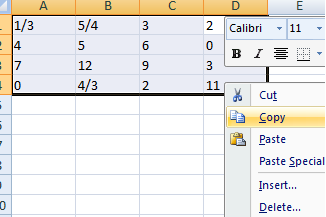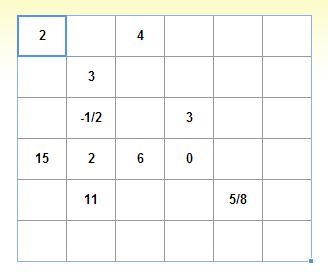Math Calculators, Lessons and Formulas

It is time to solve your math problem

mathportal.org

# Eigenvectors Calculator

This calculator computes eigenvectors of a square matrix using the characteristic polynomial. The calculator will show all steps and detailed explanation.

Eigenvectors calculator (with steps)
show help ↓↓ examples ↓↓
Input matrix
working...
examples
example 1:ex 1:
Find the eigenvectors $A = \left[ \begin{array}{cc} 0 & 1 \\ 2 & -3 \end{array} \right]$.
example 2:ex 2:
Compute the eigenvalues and eigenvectors $A = \left[ \begin{array}{cc} 3 & 1 & 4 \\ 0 & 2 & 6 \\ 0 & 0 & 5 \end{array} \right]$.
example 3:ex 3:
Find the eigenvectors of matrix $A = \left[ \begin{array}{cc} 1 & 2 & 1 \\ 6 & -1 & 0 \\ -1 & -2 & -1 \end{array} \right]$.

## How to input matrix ?

### 1: Input matrix starting from the upper left-hand corner.

 Example: To input matrix: $\left[ \begin{array}{cc} -7 & 1/4 \\ -1.3 & -2/5 \end{array} \right]$ type### 2: You don't need to enter zeros.

 Example: To input matrix: $\left[ \begin{array}{ccc} 0 & 1 & 0 \\ -1 & 0 & 0 \\ 0 & 0 & 2/3 \end{array} \right]$ type### 3: You can copy and paste matrix from excel in 3 steps.

 Step 1: Copy matrix from excel Step 2: Select upper right cell Step 3: Press Ctrl+V### 5: To delete matrix

 Select whole matrix and click deleteSearch our database of more than 200 calculators